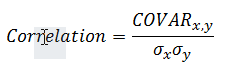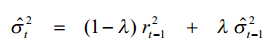# Calculating EWMA Correlation Using Excel

We had recently learned about how to estimate volatility using EWMA – Exponentially Weighted Moving Average. As we know, EWMA avoids the pitfalls of equally weighted averages as it gives more weight to the more recent observations compared to the older observations. So, if we have extreme returns in our data, as time passes, this data becomes older and gets lesser weight in our calculation. In this article we will look at how we can calculate correlation using EWMA in Excel.

We know that the correlation is calculated using the following formula:Step 1

The first step is to calculate the covariance between the two return series. We use the smoothing factor Lambda = 0.94, as used in RiskMetrics.

Consider the following equation:We use the squared returns r2 as the series x in this equation for variance forecasts and cross products of two returns as the series x in the equation for covariance forecasts. Note that the same lambda is used for all variances and covariance.

Step 2

The second step is to calculate the variances and standard deviation of each return series, as described in this article – Calculate Historical Volatility Using EWMA.

Step 3

The third step is to calculate the correlation by plugging in the values of Covariance, and Standard Deviations in the above given formula for Correlation.

Example

The following excel sheet provides an example of the correlation and volatility calculation in Excel. It takes the log returns of two stocks and calculates the correlation between them.